### Home > MC1 > Chapter 3 > Lesson 3.4.4 > Problem3-127

3-127.
1. . Si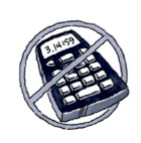mplify each expression without using a calculator. For each expression, draw a diagram or use words to explain how you know your answer makes sense. Homework Help ✎

1. −8 ÷ −4

2. 18 ÷ −3

3. −24 ÷ 2

4. 17 ÷ −1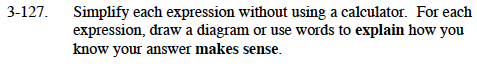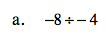Drawing a diagram will help you visualize what is happening in this expression. For this problem, we divide 8 negative integers into groups of −4.

− − − − − − − −

Since there are two groups of −4, that means that −8 divided by −4 is equal to 2.

2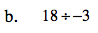Since division is the undoing of multiplication, −3 times what is equal to 18? Remember to try the problem on your own before checking the answer.

−6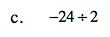Follow the steps outlined in part (a). However, it might be helpful to think of this problem as dividing −24 into 2 groups. What type of integers would be in the two groups? How many would be in each group?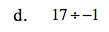This problem is similar to part (b). Try thinking of what you need to multiply by −1 to get 17.